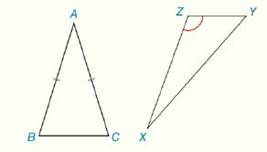Chapter 4.CR, Problem 35CR### Elementary Geometry for College St...

6th Edition
Daniel C. Alexander + 1 other
ISBN: 9781285195698

#### Solutions

Chapter
Section### Elementary Geometry for College St...

6th Edition
Daniel C. Alexander + 1 other
ISBN: 9781285195698
Textbook Problem
1 views

# Review Exercises What type of quadrilateral is formed when the triangle is reflected across the indicated side? a) Isosceles ∆ A B C across B C - b) Obtuse ∆ X Y Z across X Y -To determine

To Find:

The type of quadrilateral is formed when the isosceles ΔABC across BC¯.

Explanation

Definition:

A rhombus is a parallelogram with two congruent adjacent sides.

Properties:

1. All sides of a rhombus are congruent.

2. The diagonals of a rhombus are perpendicular.

3. The diagonals of a rhombus are perpendicular bisector of each other.

Definition:

A kite is a quadrilateral with two distinct pairs of congruent adjacent sides...

To determine

To Find:

The type of quadrilateral is formed when the Obtuse ΔXYZ across XY¯.

### Still sussing out bartleby?

Check out a sample textbook solution.

See a sample solution

#### The Solution to Your Study Problems

Bartleby provides explanations to thousands of textbook problems written by our experts, many with advanced degrees!

Get Started

#### Find more solutions based on key concepts